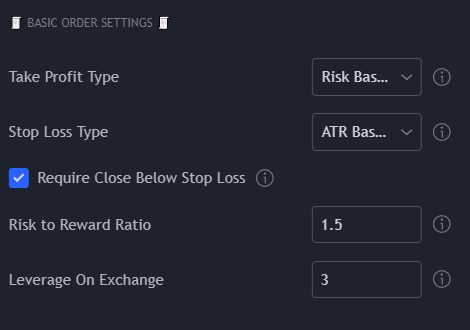Take Profit Type

What type of Take Profit calculation will be used.

• Risk Based (default) - Will use the Risk to Reward Ratio below to calculate the Take Profit Target, making it stop loss dependent. (stop loss times risk to reward ratio)

• ATR Based - Uses an independent ATR value per the ATR Settings below to determine the Take Profit Target.

• Percent Based - Uses an independent Percentage value per the Percent Based Settings below to determine the Take Profit Target.

Stop Loss Type

What type of Stop Loss calculation will be used.

• ATR Based (default) - Uses an independent ATR value per the ATR Settings below to determine the Stop Loss Limit.

• Percent Based - Uses an independent Percentage value per the Percent Based Settings below to determine the Stop Loss Limit.

Require Close Below Stop Loss

If checked will only exit orders when the closing price is below the stop loss

Risk to Reward Ratio

Only used if "Quantity Calculation Type" is set to "Risk Based".

X to 1 ratio means that if your profit target is 2, your profit target is 2 times the stop loss percentage.

Leverage On Exchange

Set to your leverage used on your exchange for your specific currency pair. This value with your equity and (when using Risk Based trading) will test if a position is possible with your requested equity. If it is not, the quantity will be adjusted to the maximum equity value in order to ensure that your bot can enter on every trade.